Courses

# Solutions to Laplace’s Equations (Part - 1) Electrical Engineering (EE) Notes | EduRev

## Electrical Engineering (EE) : Solutions to Laplace’s Equations (Part - 1) Electrical Engineering (EE) Notes | EduRev

The document Solutions to Laplace’s Equations (Part - 1) Electrical Engineering (EE) Notes | EduRev is a part of the Electrical Engineering (EE) Course Electromagnetic Theory.
All you need of Electrical Engineering (EE) at this link: Electrical Engineering (EE)

We have seen that in the region of space where there are no sources of charge, Laplace’s equation gives the potential. In this lecture, we will discuss solutions of Laplace’s equation subject to some boundary conditions.

Formal Solution in One Dimension

The solution of Laplace’s equation in one dimension gives a linear potential,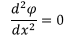has the solution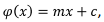where m and c are constants. The solution is featureless because it is a monotonically increasing or a decreasing function of x. Further, since the potential at x is the average of the potentials at x+a and x-a, it has no minimum or maximum.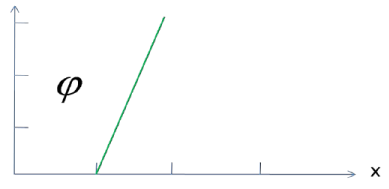Formal Solution in Two Dimension

In two dimensions , the Laplace’s equation is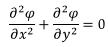Since the derivatives are partial, we must have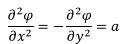where a is a constant. Thus the solution to the equation is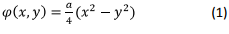Before looking at this solution let us look at a closely resembling Poisson’s equation in 2 dimensions,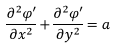which has the solution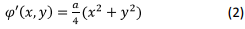The solution looks like cup having a minimum at x = 0, y = 0.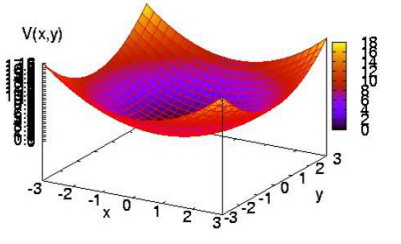On the other hand, if you look at the solution (1) of the Laplace’s equation, the graph of the potential as a function of x and y looks like the following :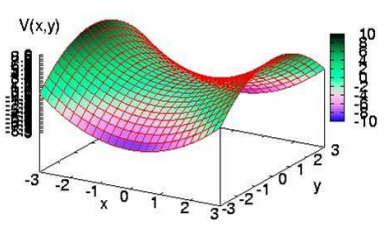Note that the function does not have a minimum or a maximum. The point x=0, y=0 is a “saddle point” where the function rises in one direction and decreases in another direction, much like a saddle on a horse where the seat slopes downwards along the back but rises along its length both forward and backward.

[Mathematically, in two dimensions, if a function f partial derivatives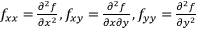such that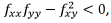the function has a saddle point. If the expression has a positive value, the function has a maximum or a minimum depending on whether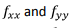are both negative or both positive respectively. For eqn. (1) at the point (0,0)the expression is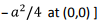This is true in any dimension because for a minimum the second partial derivative with respect to each variable must be negative so that their sum cannot add to zero as is required by Laplace’s equation.

Earnshaw’s Theorem

A consequence of solutions Laplace’s equation not having a minimum is that a charge cannot be held in equilibrium by electrostatic forces alone (i.e., if we are to keep a charge in equilibrium, we need forces other than just electrostatic forces; or for that matter, magnetostatic or static gravitational forces). This is known as the Earnshaw’s theorem.

The proof is elementary. In a region of space containing no charge, Laplace’s equation is valid for the potential φ If a charge is to be kept in this potential, its potential energy qφ also satisfies the Laplace’s equation. Since the solutions of Laplace’s equation do not have minima, the charge cannot be in static equilibrium.

In the remaining part of this lecture we will discuss the solutions of Laplace’s equation in three dimensions in different coordinate systems.

Solution in Cartesian Coordinates :

The Laplace’s equation is Cartesian coordinates is given by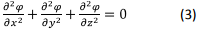We will look into the solution of this equation in a region defined by a parallelepiped of dimensions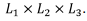In principle, one could choose boundary conditions such as prescribing the potential on each of the six surfaces of the parallelepiped. However, we consider a situation in which five walls of the parallelepiped are conductors which are joined together so that each is maintained at the same potential, which we take to be zero. The sixth plate of the parallelepiped at z = L3 is made with a different sheet of metal and is kept at a potential which is a known function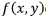of position on the plate.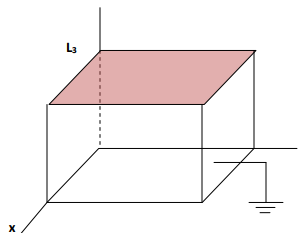Summarizing the boundary conditions, we have,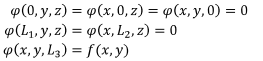We will use a technique known as separation of variables to solve the equation. We assume that the solution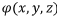can be written as a product of three independent functions, X, Y and Z, the first one depending on the variable x alone, the second on variable y alone and the third on the variable z alone. It is not that such a trick will always work, but in cases where it does, the uniqueness theorem would guarantee us that that would be the only solution. Keeping this in view, let us attempt such a solution: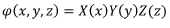Substituting this in eqn. (3) and dividing both sides by the product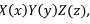we get,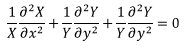In this equation there are three terms, the first depending on x alone, the second on y alone and the third on z alone. Since x,y,z are arbitrary, the equation can be satisfied only if each of the terms is a constant and the three constants are such that they add up to zero.

Let us write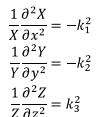Where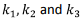are constants, which satisfy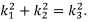The boundary conditions on five faces of the parallelepiped where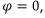can be written as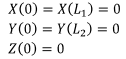However, on the sixth face we must have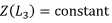because the potential on this face depends only on x,y whereas Z can only depend on z.

Consider the first equation, viz.,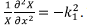The solution of this equation is well known to be linear combination of sine and cosine functions. Keeping the boundary conditions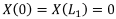in mind, we can write the solution to be given by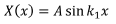where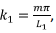where m is an integer not equal to zero (as it would make the function identically vanish) and A is a constant .

In a very similar way the solution for Y is written as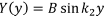With B being a constant and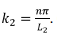Once again n is a non-zero integer.

The solution for Z is a little more complicated because of the constraint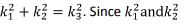are positive integers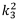is also positive.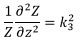Then has solutions which are hyperbolic functions,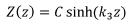with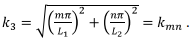Thus, we can write the expression for the potential as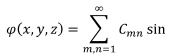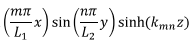The solution above satisfy the first five boundary conditions. Let us see the effect of the last boundary condition.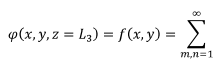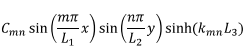The constants Cmn can be derived from above equation because f(x,y) is a known function. For this we use the orthogonal property of the trigonometric functions,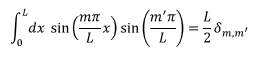This gives,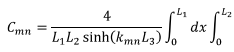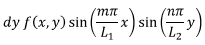Take for instance,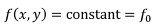The integral above is then product of two integrals,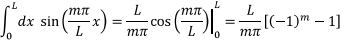Thus Cmn is non-zero only when both m and n are odd and has the value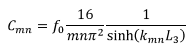In the next lecture we will obtain solutions of Laplace’s equation in spherical coordinates.

Tutorial Assignment

1. Find an expression for the potential in the region between two infinite parallel planes z = 0 and z = 1 the potential on the planes being given by the following :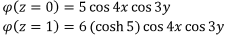2. Obtain a solution to Laplace’s equation in two dimensions in Cartesian coordinates assuming that the principle of variable separation holds.

3. Find the solution of two dimensional Laplace’s equation inside a rectangular region bounded by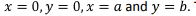The potential has value zero on the first three boundaries and takes value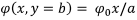on the fourth side.

Solution to Tutorial Assignment

1. We know that the general solution is a product of linear combination of either sine, cosine or hyperbolic sine and hyperbolic cosine functions with a relationship between the arguments. . In this case, since on both plates the dependence on x and y is given as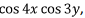the general solution is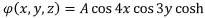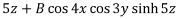Where the argument of the hyperbolic function is obtained from the relation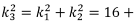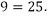Substituting the boundary condition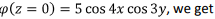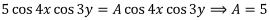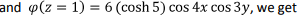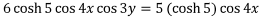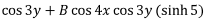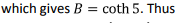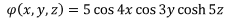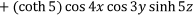2. We start with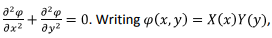we get, on substituting and dividing throughout by XY,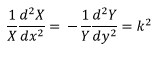The solution is then a product of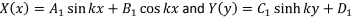cosh ky. We can reduce the number of constants from 5 to 4 by redefining the constants. For instance, if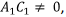we define the constants as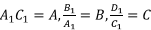and write,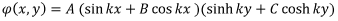The constants A, B, C and k are to be determined from boundary conditions.

3. We have seen that the general solution is a product of linear combination of sine and cosine in one of the variables and hyperbolic sine and cosine in the other variable. Since the potential is to be zero for x = a for all values of y, this is possible only if we choose the solution for the variable x to be the trigonometric function and for y to be hyperbolic function. This is because a sine function can become zero at values of its argument other than zero, a hyperbolic function cannot. Further, only sine and sinh functions need to be considered so that at x= 0 and at y=0, the function vanishes. Thus we write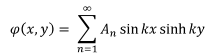In this form, each term of the series automatically satisfies the boundary conditions at x= 0 and at y=0. Since the potential is to become zero for all values of y for x= 1, this is possible if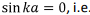if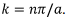Thus the potential function becomes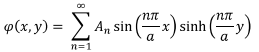We have to satisfy the only remaining boundary condition, viz., for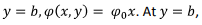we have,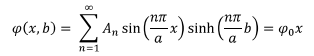To determine the coefficients An, we multiply both sides of the expression by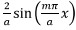and integrate over x from 0 to a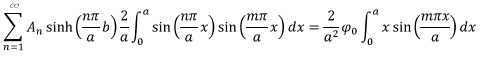The integral on the left gives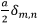while that on the right gives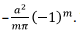Thus,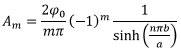This completely determines the potential.

Self Assessment Quiz

1. Does the function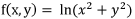satisfy Laplace’s equation? 2. Find an expression for the potential in the region between two infinite

2. Find an expression for the potential in the region between two infinite parallel planes z = 0 and z π/2 on which the potentials are respectively given by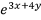and 0.

3. Find an expression for the potential in the region between two infinite parallel planes, the potential on which are given by the following :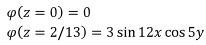Solution to self assessment Quiz

1. Yes, a direct calculation shows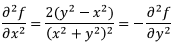2. In this case also we have solutions which are written as products of separate variables. The solution can be written as (since X and Y are hyperbolic functions, the function Z is a linear combination of sine and cosine),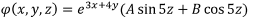The constants A and B can be determined by the boundary conditions. For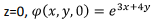gives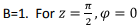gives A=0. Thus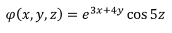3. The z dependence of the potential is a linear combination of hyperbolic sine and cosine function with argument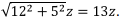Thus the general expression is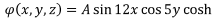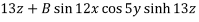For z=0, the potential being zero, the cosh term vanishes,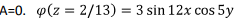gives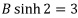so that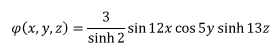Offer running on EduRev: Apply code STAYHOME200 to get INR 200 off on our premium plan EduRev Infinity!

,

,

,

,

,

,

,

,

,

,

,

,

,

,

,

,

,

,

,

,

,

;